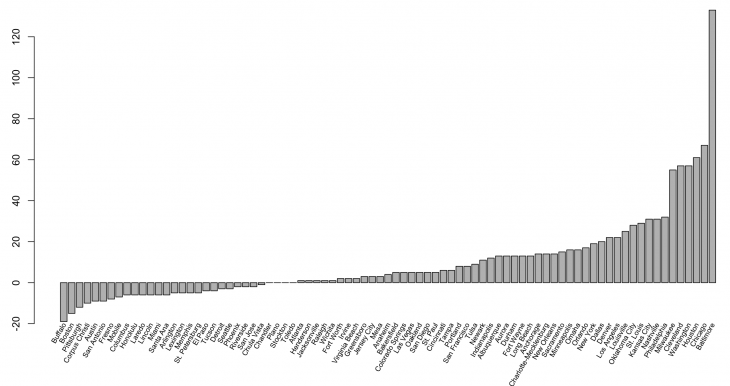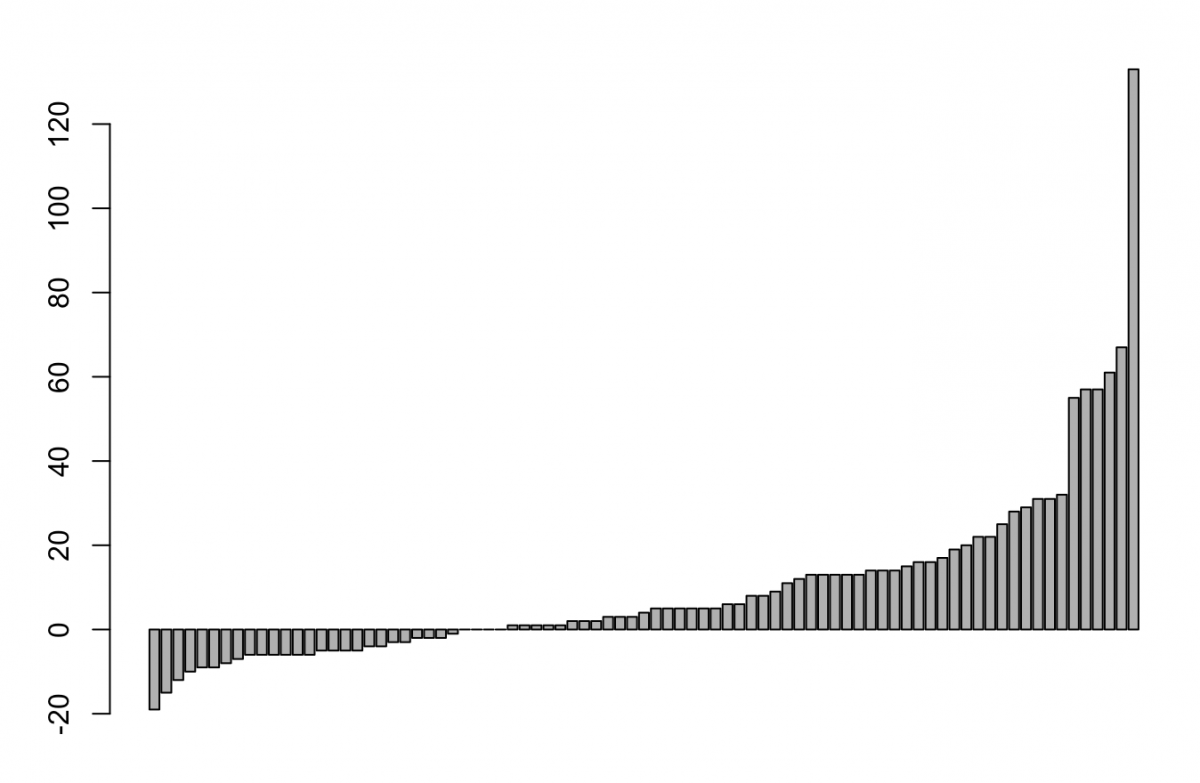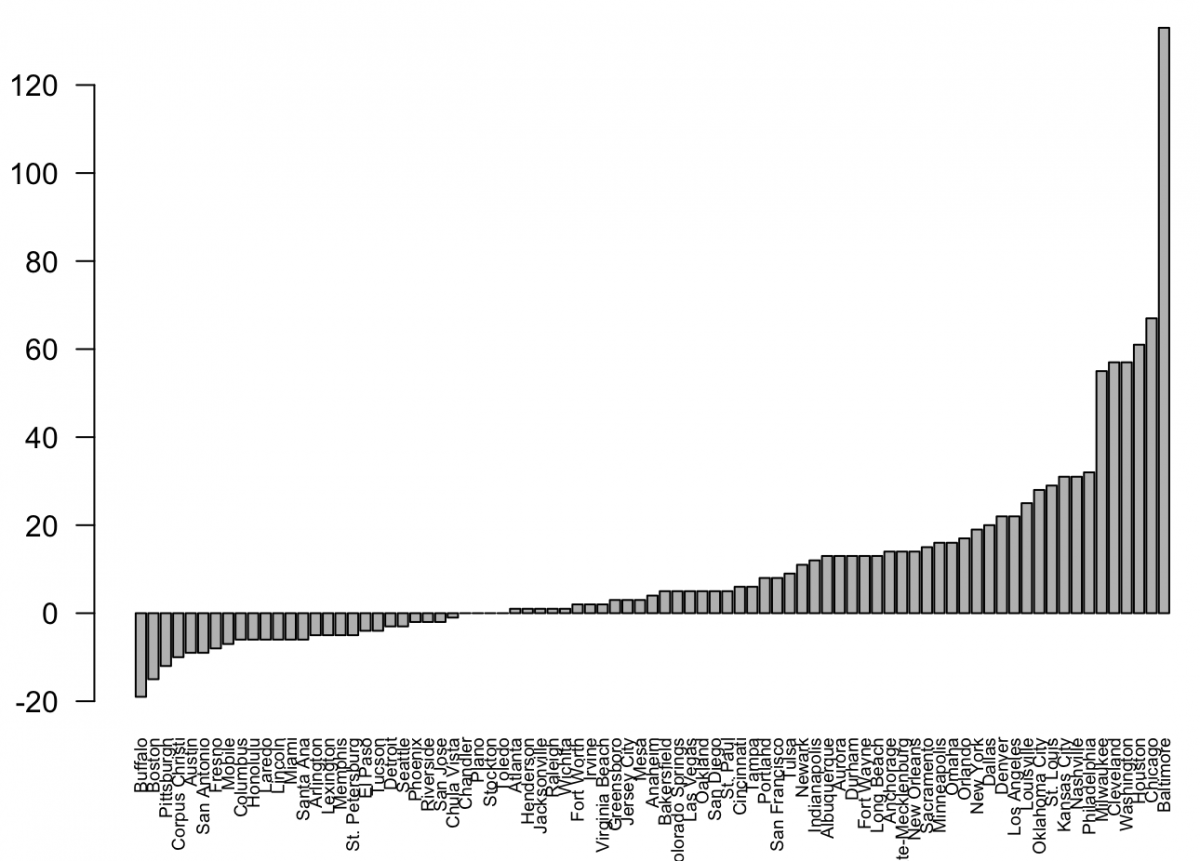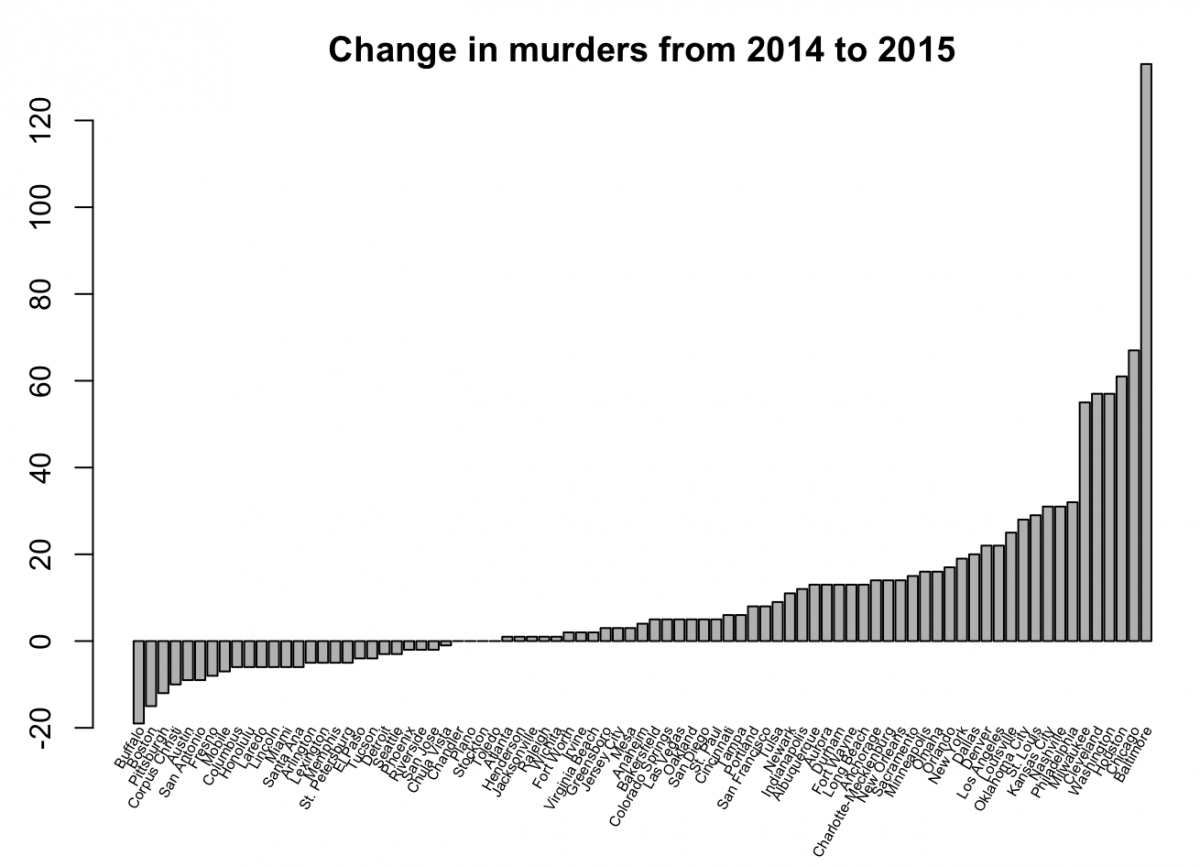# How to create a barplot in R

In playing with the `fivethirtyeight` R package for another Storybench tutorial, we learned some basics of plotting a bar chart in R using data from a csv. Below, we’ve outlined the steps we’ve taken to create a barplot in R using murders_final_sort.csv, cleaned and created in this tutorial.

In RStudio, load the csv file.

``murders_final_sort <- read.csv(file="murders_final_sort.csv")``

Read the column names in RStudio’s console with `names(murders_final_sort)`.

## Create a simple barplot

Using `barplot(murders_final_sort\$change)` you can plot the “change” column. Run the script and you’ll see this:## Extend the y-axis

To extend the y-axis to -20, add:

``````barplot(murders_final_sort\$change,
ylim = c(-20, 120))``````Finally, we’ll add some labels. There are many different ways to add labels in R. Let’s start with simple labels, added with `names.arg`, whose font is made smaller with `cex.names`. They are made perpendicular with `las = 2`.

``````barplot(murders_final_sort\$change,
ylim = c(-20, 120),
names.arg = murders_final_sort\$city,
las = 2,
cex.names = 0.6)``````## Add a title and place labels at 60 degrees

The following code gets a little complicated and there are several other ways to achieve this end result. Notice `srt = 60` places the labels at 60 degrees while `y = -20` and `offset = -0.1` help move the labels around. Finally, `pos = 2` justifies them right. To read more about those, go here.

``````midpts <- barplot(murders_final_sort\$change,
cex.axis = 1.0, cex.names = 0.6,
ylim = c(-20, 120), main = "Change in murders from 2014 to 2015"
)

text(murders_final_sort\$city,
x = midpts,
offset = -0.1,
y = -20,
cex = 0.5,
srt = 60,
xpd = TRUE,
pos = 2 )
``````

The final barplot should look like this:Latest posts by Aleszu Bajak (see all)

#### One thought on “How to create a barplot in R”

•Janet says:

Thanks for the information, it’s very great.
Please can you help me with how to create a grouped bar chart using a CSV file?